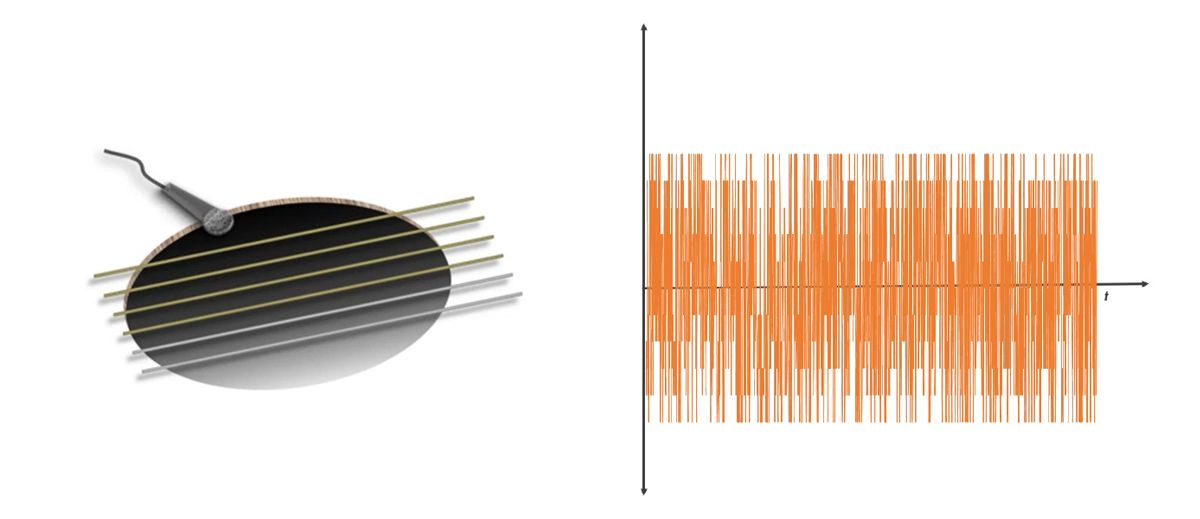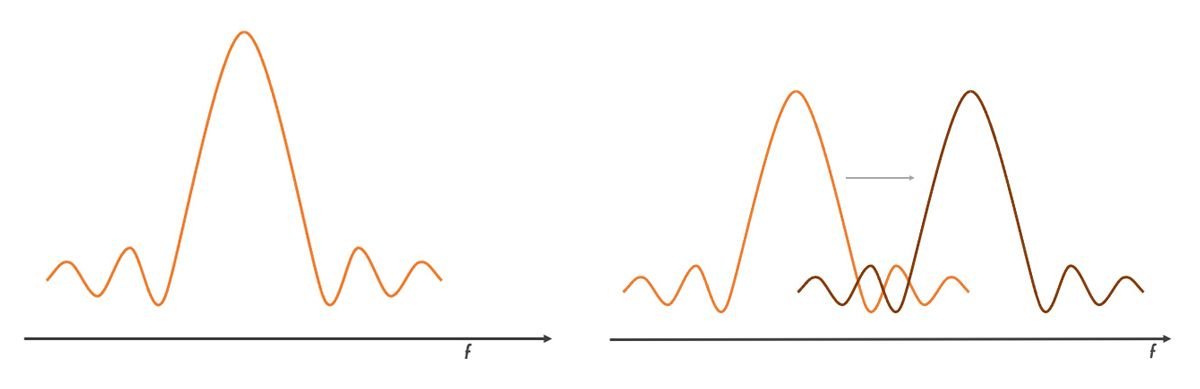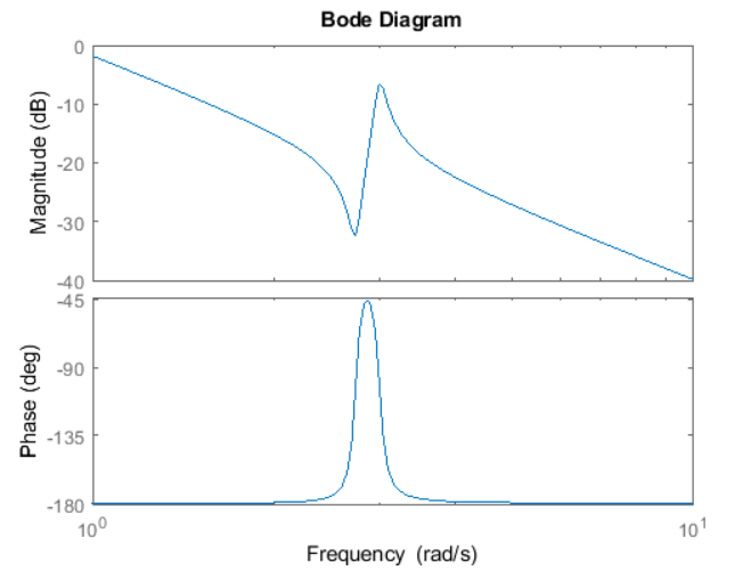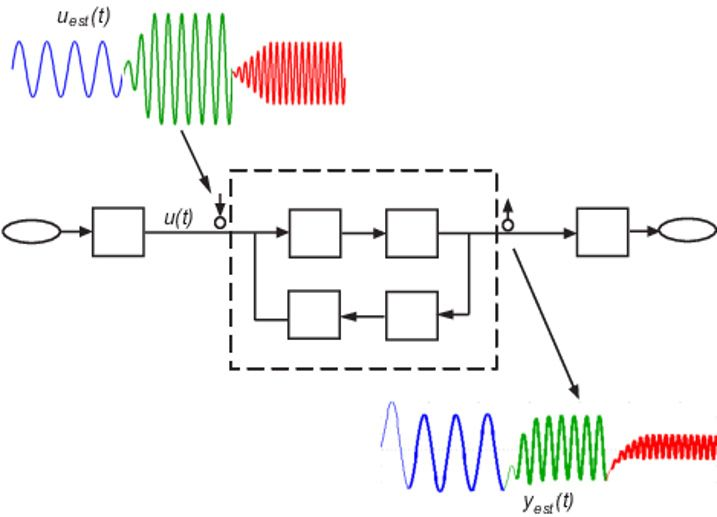# 频率响应概述### 获取系统的频率响应

1. 如果系统具备传递函数或状态空间模型形式的线性表示，则可以使用以下三种图之一来绘制频率响应：波特图奈奎斯特图尼柯尔斯图。波特图将幅值和相位表示为激励信号频率的函数（图 4）。
2. 例如，假设系统 $$(H)$$ 有如下传递函数表示：

$$H(s) = {s^2+ 0.1s + 7.5\over s^4+0.12s^3+9s^2}.$$

您可以使用以下命令在 MATLAB 中绘制其频率响应：

$$H = {tf([1 \quad 0.1\quad 7.5], [1 \quad 0.12 \quad 9 \quad 0 \quad 0]});$$

$$bode(H)$$1. 此时，如果您可以访问物理系统的输入输出测试数据，则可以通过 System Identification Toolbox™ 使用数据驱动的建模方法来识别系统的传递函数、状态空间表示和频率响应模型。1 至 5 星（由低到高）

PRBS 离线 宽带 ★★ ★★★ 系统包含高频开关组件，如通信和电力电子系统。